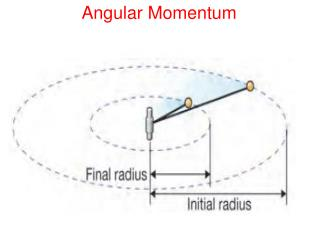DownloadDownload PresentationAngular Momentum

# Angular Momentum

Télécharger la présentation## Angular Momentum

- - - - - - - - - - - - - - - - - - - - - - - - - - - E N D - - - - - - - - - - - - - - - - - - - - - - - - - - -
##### Presentation Transcript

1. Angular Momentum

2. Angular Momentum • MOMENTUM RESULTING FROM AN OBJECT MOVING IN LINEAR MOTION IS CALLED LINEAR MOMENTUM. • MOMENTUM RESULTING FROM THE ROTATION (OR SPIN) OF AN OBJECT IS CALLED ANGULAR MOMENTUM.

3. Conservation of Angular Momentum • ANGULAR MOMENTUM IS IMPORTANT BECAUSE IT OBEYS A CONSERVATION LAW, AS DOES LINEAR MOMENTUM. • THE TOTAL ANGULAR MOMENTUM OF A CLOSED SYSTEM STAYS THE SAME.

4. L = Iw Calculating angular momentum ANGULAR MOMENTUM IS CALCULATED IN A SIMILAR WAY TO LINEAR MOMENTUM, EXCEPT THE MASS AND VELOCITY ARE REPLACED BY THE MOMENT OF INERTIA AND ANGULAR VELOCITY. Moment of inertia (kg m2) ANGULAR MOMENTUM (KG M/SEC2) Angular velocity (rad/sec)

5. Calculating angular momentum • You are asked for angular momentum. • You are given mass, shape, and angular velocity. • Hint: both rotate about y axis. • Use L= I, Ihoop = mr2, Ibar = 1/12 ml2 An artist is making a moving metal sculpture. She takes two identical 1 kg metal bars and bends one into a hoop with a radius of 0.16 m. The hoop spins like a wheel. The other bar is left straight with a length of 1 meter. The straight bar spins around its center. Both have an angular velocity of 1 rad/sec. Calculate the angular momentum of each and decide which would be harder to stop.

6. TORQUE

7. WHEN QUARTERBACKS THROW THE FOOTBALL, THEY IMPART A SPIN WITH THEIR FINGERS, SO THAT THE BALL SPINS RAPIDLY AS IT FLIES THROUGH THE AIR. FOOTBALL FANS CALL A GOOD PASS A TIGHT SPIRAL.

8. BULLETS WHICH COME OUT OF A RIFLED BARREL HAVE GROOVES CUT INTO THEM, LIKE THIS ONE. THESE LEAVE BALLISTIC FINGER PRINTS

9. ANGULAR MOMENTUM

10. Calculating angular momentum • Solve hoop: Ihoop= (1 kg) (0.16 m)2 = 0.026 kg m2 • Lhoop= (1 rad/s) (0.026 kg m2) = 0.026 kg m2/s • Solve bar: Ibar= (1/12)(1 kg) (1 m)2 = 0.083 kg m2 • Lbar = (1 rad/s) (0.083 kg m2) = 0.083 kg m2/s • The bar has more than 3x the angular momentum of the hoop, so it is harder to stop.

11. 12.3 Gyroscopes angular momentum • A gyroscope is a device that contains a spinning object with a lot of angular momentum. • Gyroscopes can do amazing tricks because they conserve angular momentum. • For example, a spinning gyroscope can easily balance on a pencil point.

12. 12.3 Gyroscopes angular momentum • A gyroscope on the space shuttle is mounted at the center of mass, allowing a computer to measure rotation of the spacecraft in three dimensions. • An on-board computer is able to accurately measure the rotation of the shuttle and maintain its orientation in space.